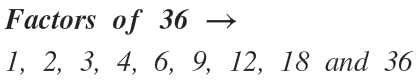Select Page

# Factors of 36

The number 36 has 9 factors and is a composite number.

1. To calculate the factors of 36 we divide, whenever we can, among all the numbers that are less than it.

36:1=36
36:2=18
36:3=12
36:4=9
36:6=6
36:9=4
36:12=3
36:18=2
36:36=1### Factors of 35

The number 35 has 4 factors and is a composite number. To calculate the factors of 35 we divide,...

### Factors of 34

The number 4 has 4 factors and is a composite number. To calculate the factors of 34 we divide,...

### Factors of 33

The number 33 has 4 factors and is a composiite number. To calculate the factors of 33 we divide,...

### Factors of 32

The number 32 has 6 factors and is a composite number. To calculate the factors of 32 we divide,...

### Factors of 31

The number 31 has 2 factors and is a prime number. To calculate the factors of 31 we divide,...

### Factors of 30

The number 30 has 8 factors and is a composite number. To calculate the factors of 30 we divide,...

### Factors of 29

The number 29 has 2 factors and is a prime number. To calculate the factors of 29 we divide,...

### Factors of 28

The number 28 has 5 factors and is a composite number. To calculate the factors of 28 we divide,...

### Factors of 27

The number 27 has 4 factors and is a composite number. To calculate the factors of 27 we divide,...

### Factors of 26

The number 26 has 4 factors and is a composite number. To calculate the factors of 26 we divide,...